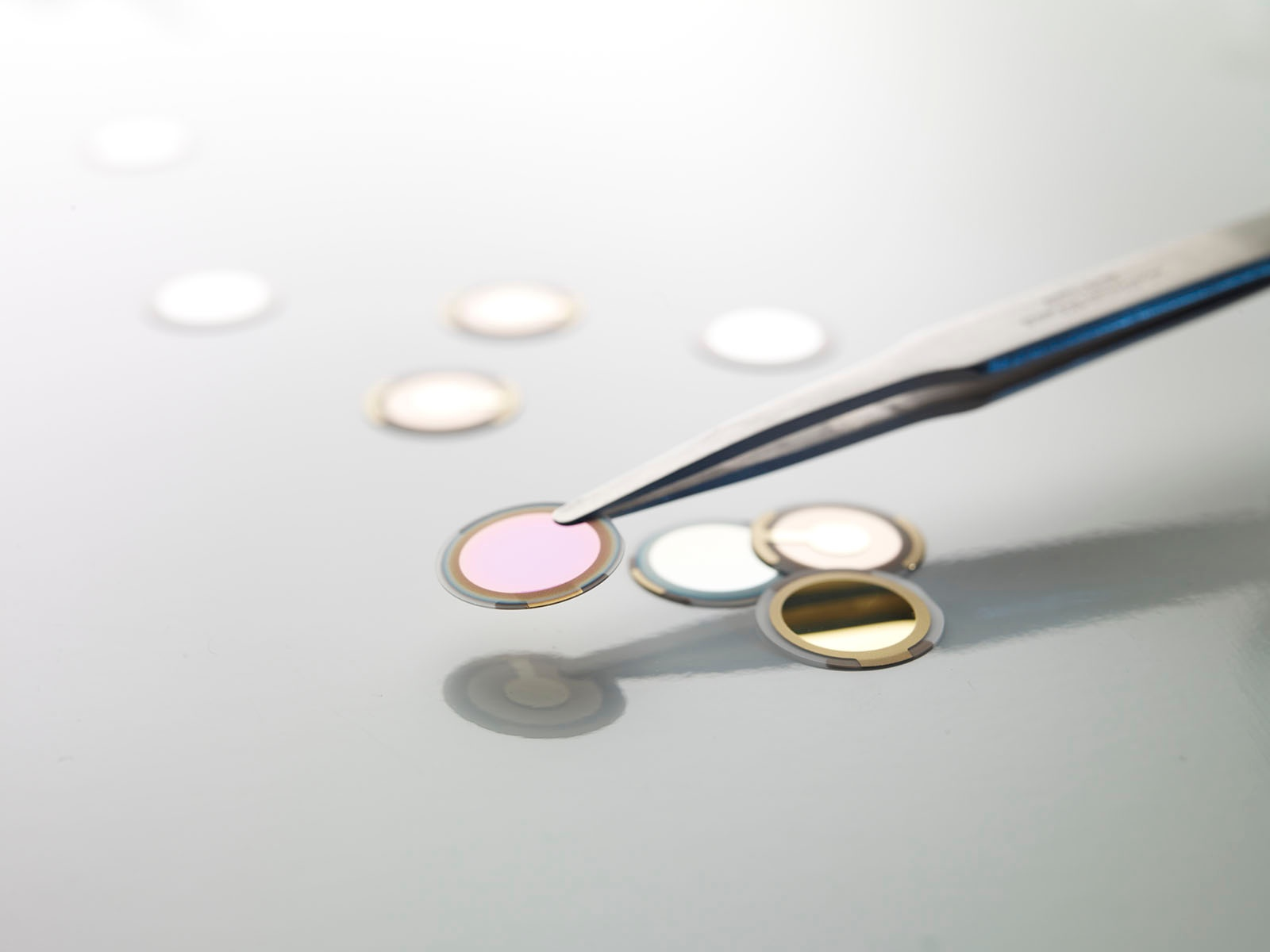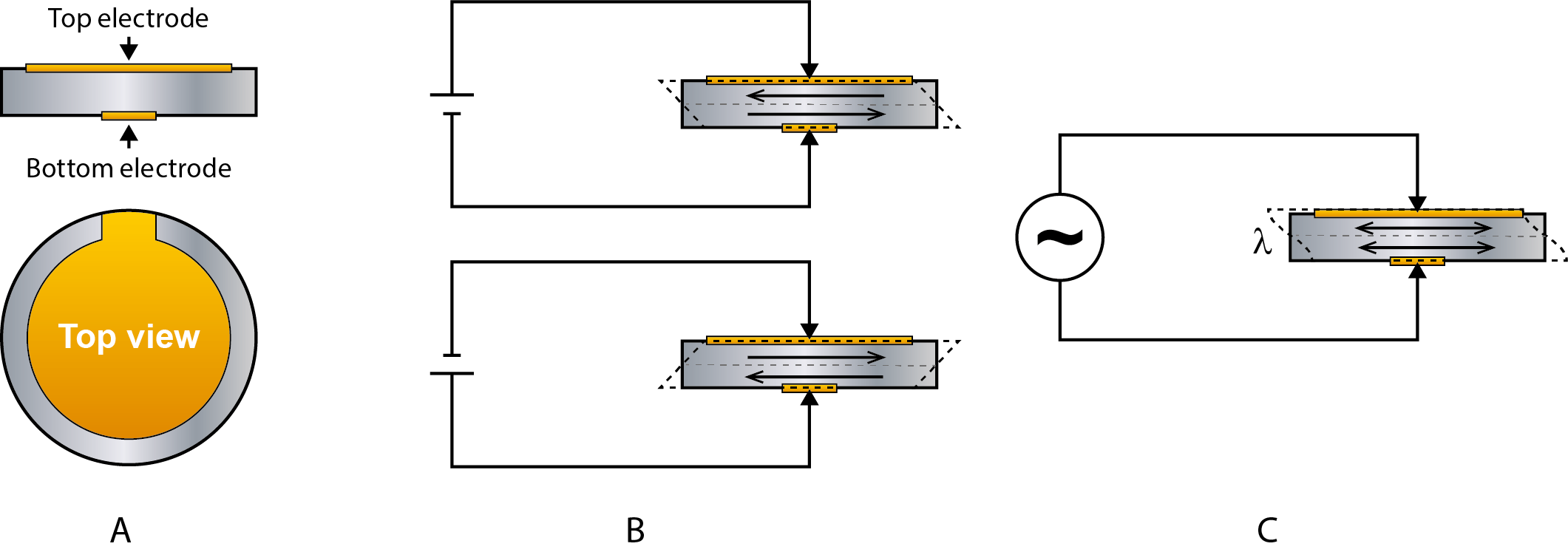Malin Edvardsson Apr 9, ’19 > 4 min

# How does QCM-technology work?

## Surface Science Blog

The quartz crystal microbalance, or QCM as it is usually called, has been around since the 60’ where it has been used for monitoring of thin-film deposition and characterization of thin films. So how does this technology work?

## Piezoelectricity and the QCM working principle

The core of the QCM technology is the quartz crystal. The QCM sensor is made of a thin quartz crystal disk sandwiched between two electrodes, Fig. 1A. Quartz is a piezoelectric material, i.e. a material where a mechanical deformation gives rise to a movement of charge in the material and vice versa, i.e. an applied electrical field will give rise to a mechanical deformation. Practically speaking, if a voltage is applied over the QCM sensor electrodes it will change shape. How it will change shape depends on how the crystal is cut and the direction of the voltage. Quartz crystals used in QCM applications are cut in such a way that the resulting deformation shears the disk in the thickness direction as indicated in Fig. 1B. If an alternating voltage is applied, the disk will oscillate back and forth in synch with the applied voltage, Fig. 1C.Figure 1. A.) Schematic side and top views of a QCM sensor. The QCM sensor is made of a quartz crystal disk, sandwiched between two metal electrodes. B.) The application of a voltage over the disk will make it deform. The direction of the deformation depends on the sign of the applied voltage and the crystal cut. C.) When an alternating voltage is applied to the quartz crystal it will oscillate back and forth.

## The resonance frequency depends on the thickness of the QCM sensor

At a certain frequency, f, the oscillating disk will be in resonance. The resonance condition is described in Eq. 1, and is a function of the thickness, h, of the disk. The parameters λ, υq and are the wavelength, speed of sound in quartz and overtone number respectively.

f =  n·υq/λ = n·υq/(2h)                                      (1)

Looking at this equation, we see that the thicker the disk, the lower the resonance frequency. Therefore, information about the resonance frequency can reveal the disk thickness. Based on this, a relation was formulated by Günter Sauerbrey in 1959, the so called Sauerbrey equation. The relation says that if the sensor disk is loaded with a thin and rigid film of some other material, where the film is tightly coupled to the oscillating sensor, there is a proportional change in the sensor’s resonant frequency. I.e. as mass is added or removed from an oscillating sensor, there will be a corresponding frequency change. Mass or thickness changes can hence be revealed via measurements of the resonance frequency, and the QCM technology was born.

## QCM technology relates frequency change to mass change

To conclude, the QCM, which is a real-time, surface sensitive technique, monitors the change in mass or thickness of layers adhering to the surface of a quartz crystal. This is achieved by measuring the change in resonance frequency of the quartz crystal upon excitation by a driving voltage.1 In certain cases, the mass change can be calculated with an equation called the Sauerbrey equation, which is derived from eq. 1.

## Explore the blog

You have only scratched the surface.